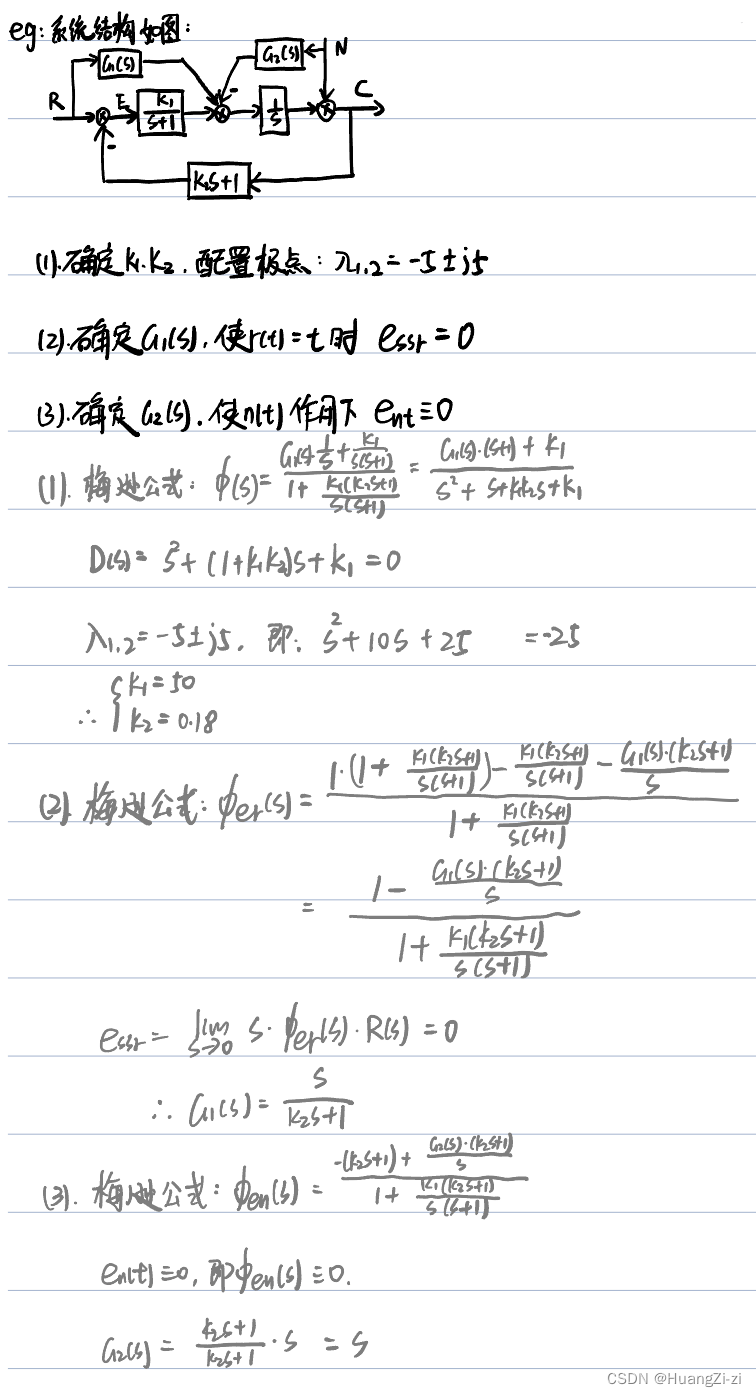## 「自控原理」3.3 稳定性与稳态误差、时域校正

news/2023/6/8 0:26:35

### 文章目录

• 稳定性分析
• 稳定的充要条件与必要条件
• 劳斯判据-Routh
• 例题
• 两种特殊情况
• 问题辨析
• 稳态误差
• 误差与稳态误差的定义
• 计算稳态误差的一般方法
• 静态误差系数法
• 动态误差系数法
• 扰动作用下的稳态误差
• 时域校正
• 反馈校正
• 复合校正

# 稳定性分析

## 稳定的充要条件与必要条件

lim⁡t→∞k(t)=0\lim \limits_{t\rightarrow \infty}k(t)=0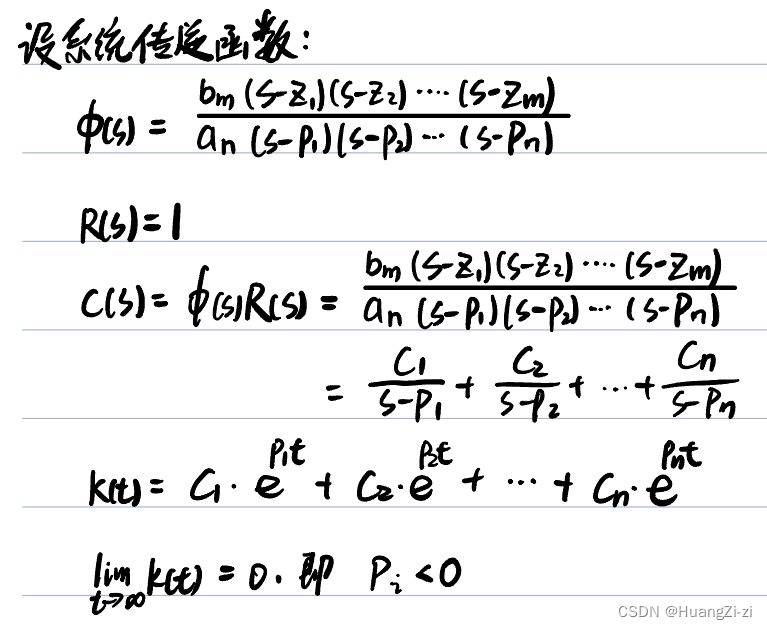cic_is=−pis=-p_i处的留数。因此lim⁡t→∞k(t)=0\lim \limits_{t\rightarrow \infty}k(t)=0的充要条件是：特征根具有负实部，也就是系统的闭环极点全部位于左半s平面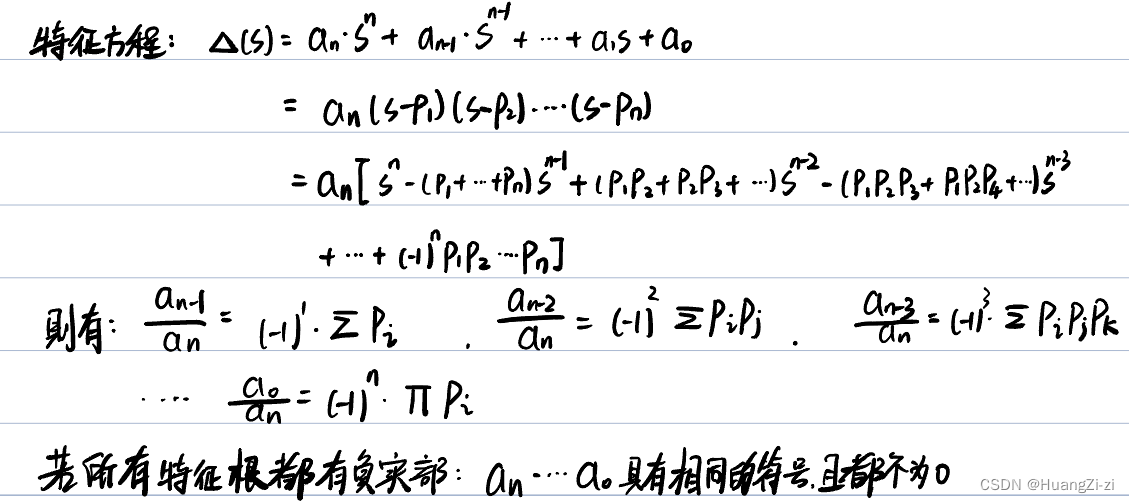## 劳斯判据-Routh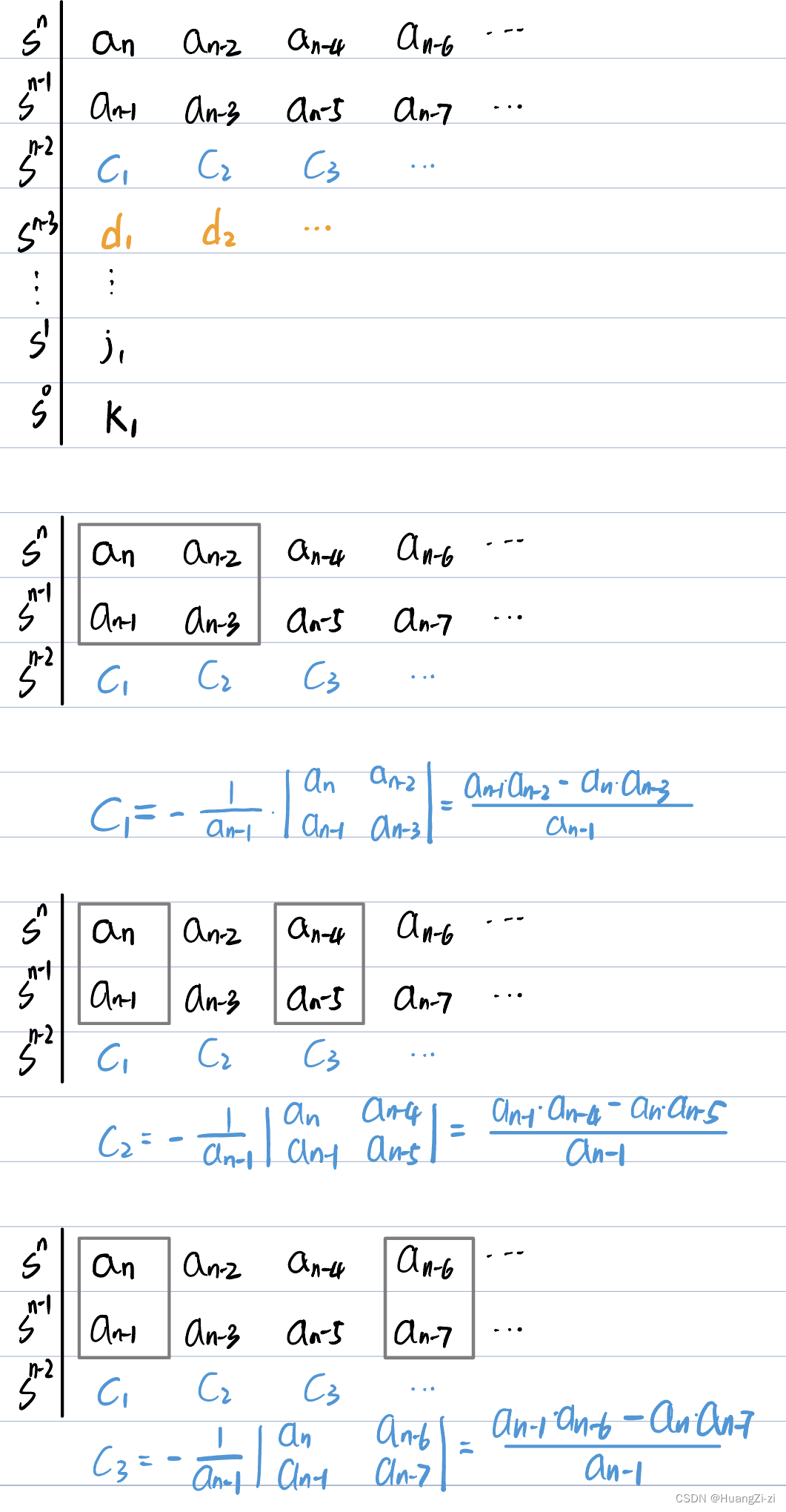−1第x−1行的第一个元素⋅第x−1和x−2行的第1和第i+1个元素组成的行列式-\frac{1}{第x-1行的第一个元素}\cdot 第x-1和x-2行的第1和第i+1个元素组成的行列式

### 例题

1. 用劳斯判据判断系统是否稳定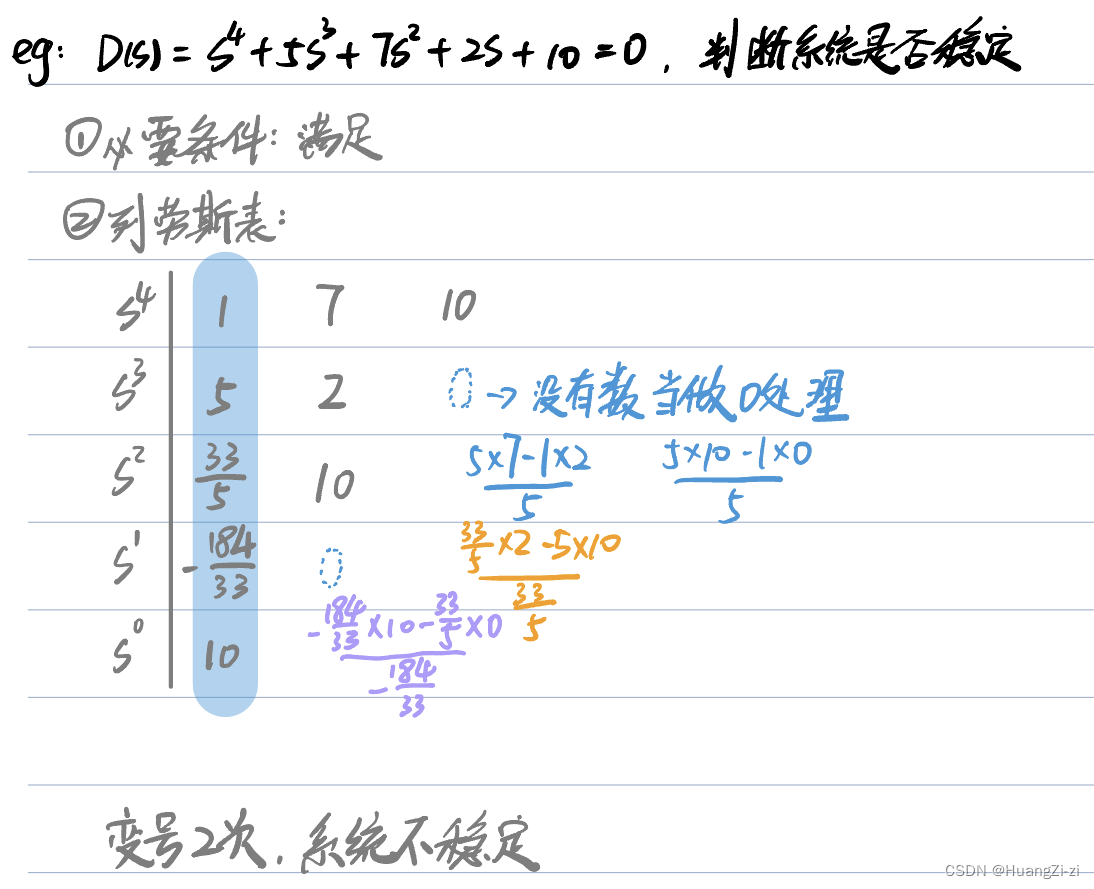判断稳定性的题，如果没有特殊要求一定先看是否满足必要条件。如果过不满足那么可以直接结论不稳定。
2. 用劳斯判据确定参数范围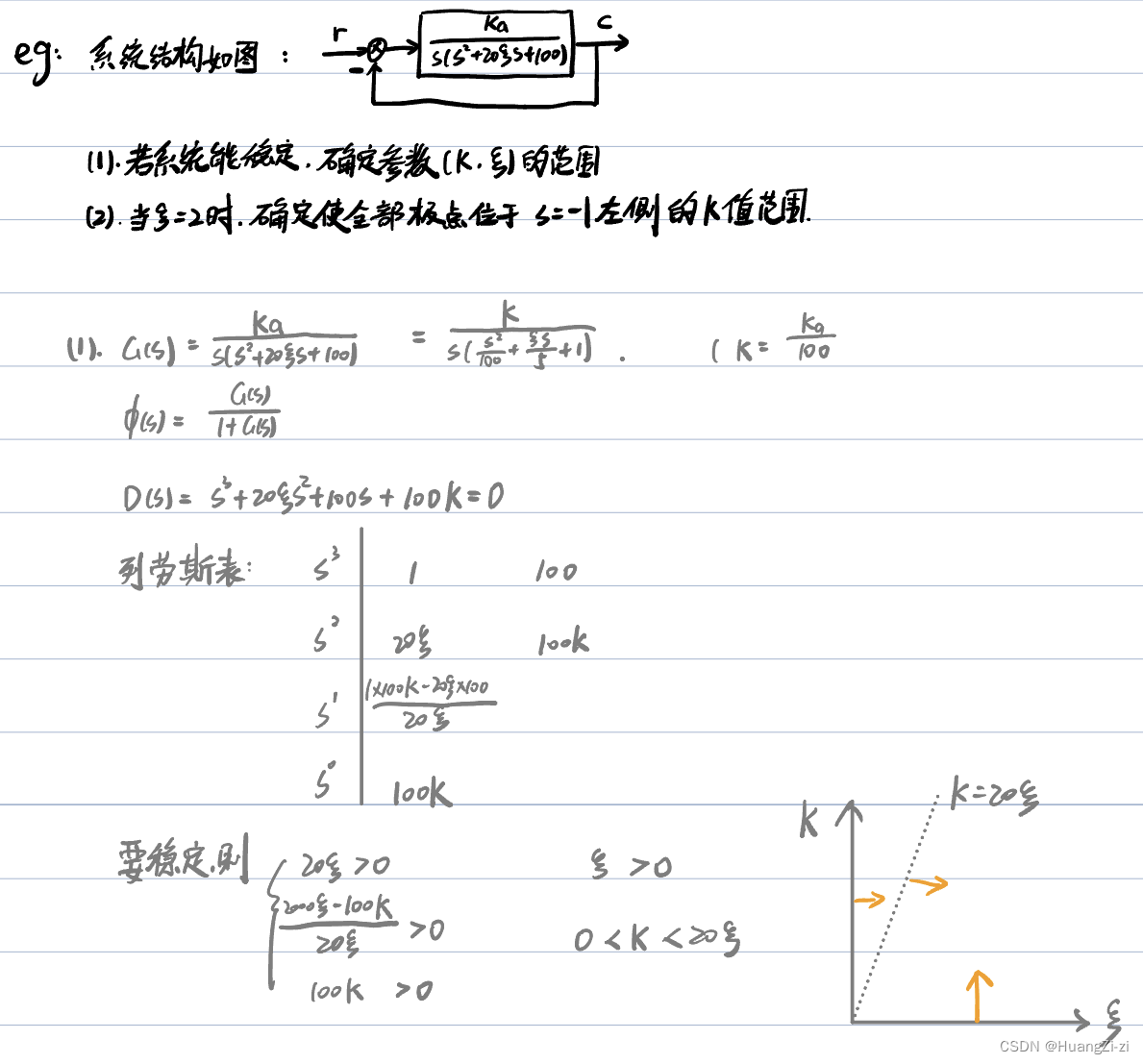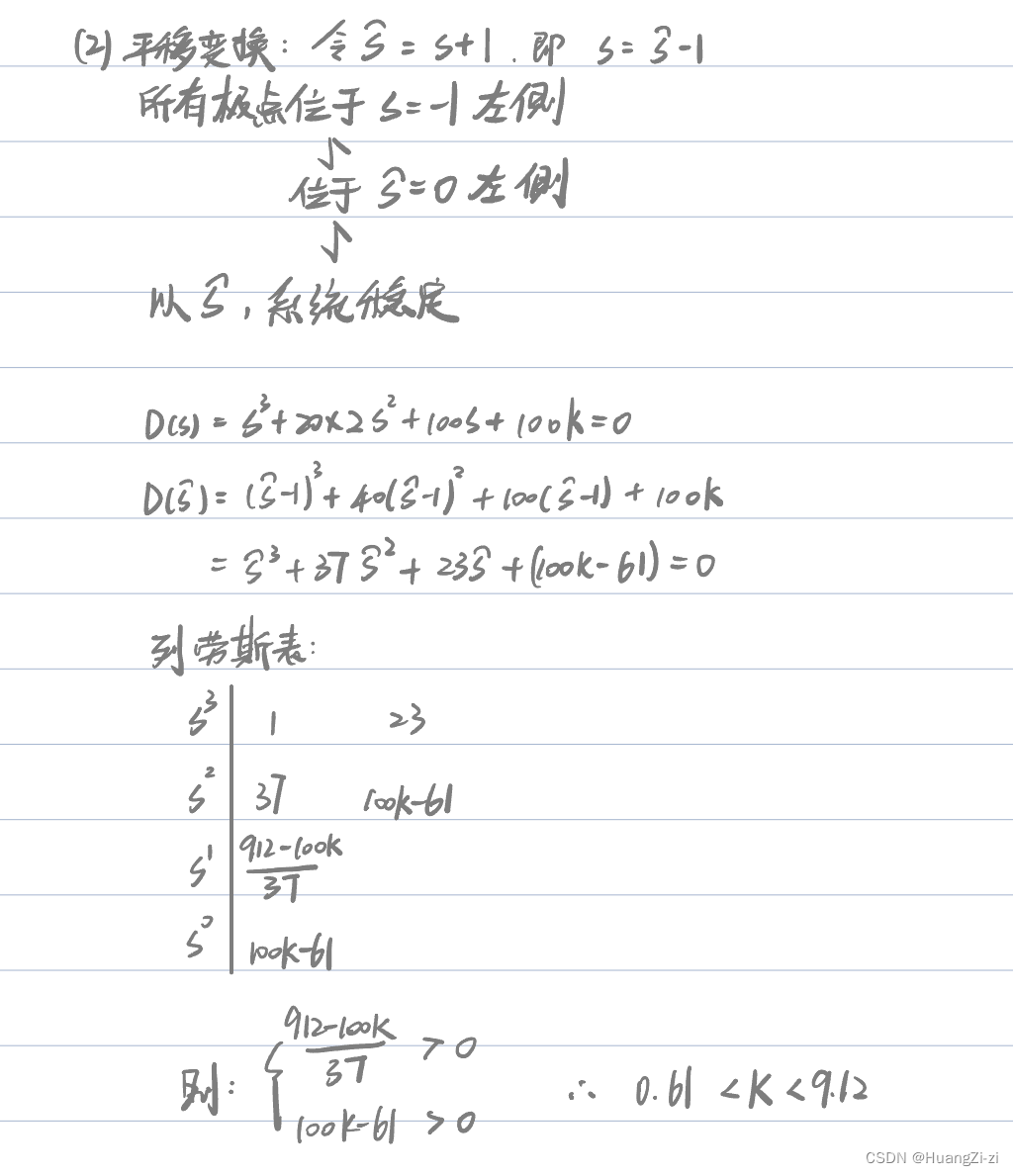### 两种特殊情况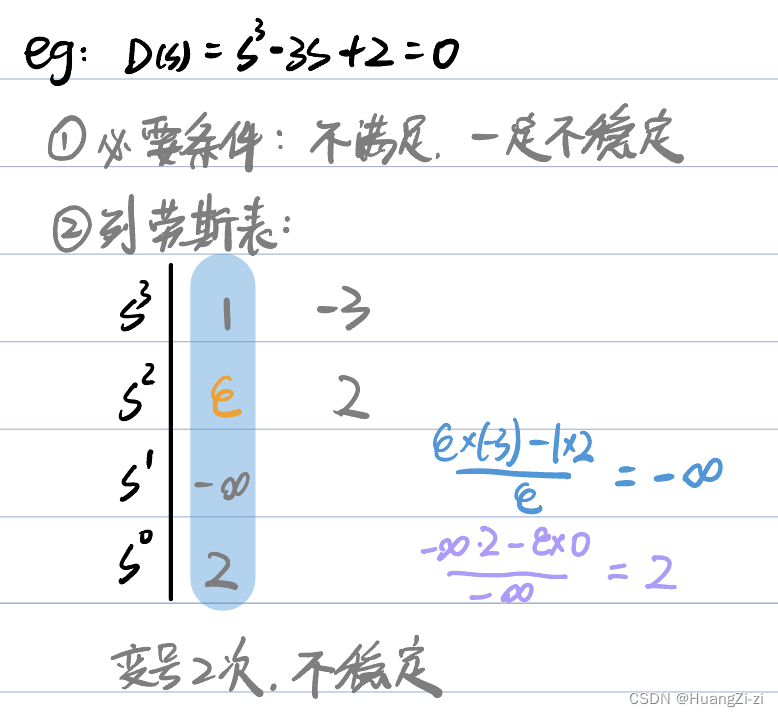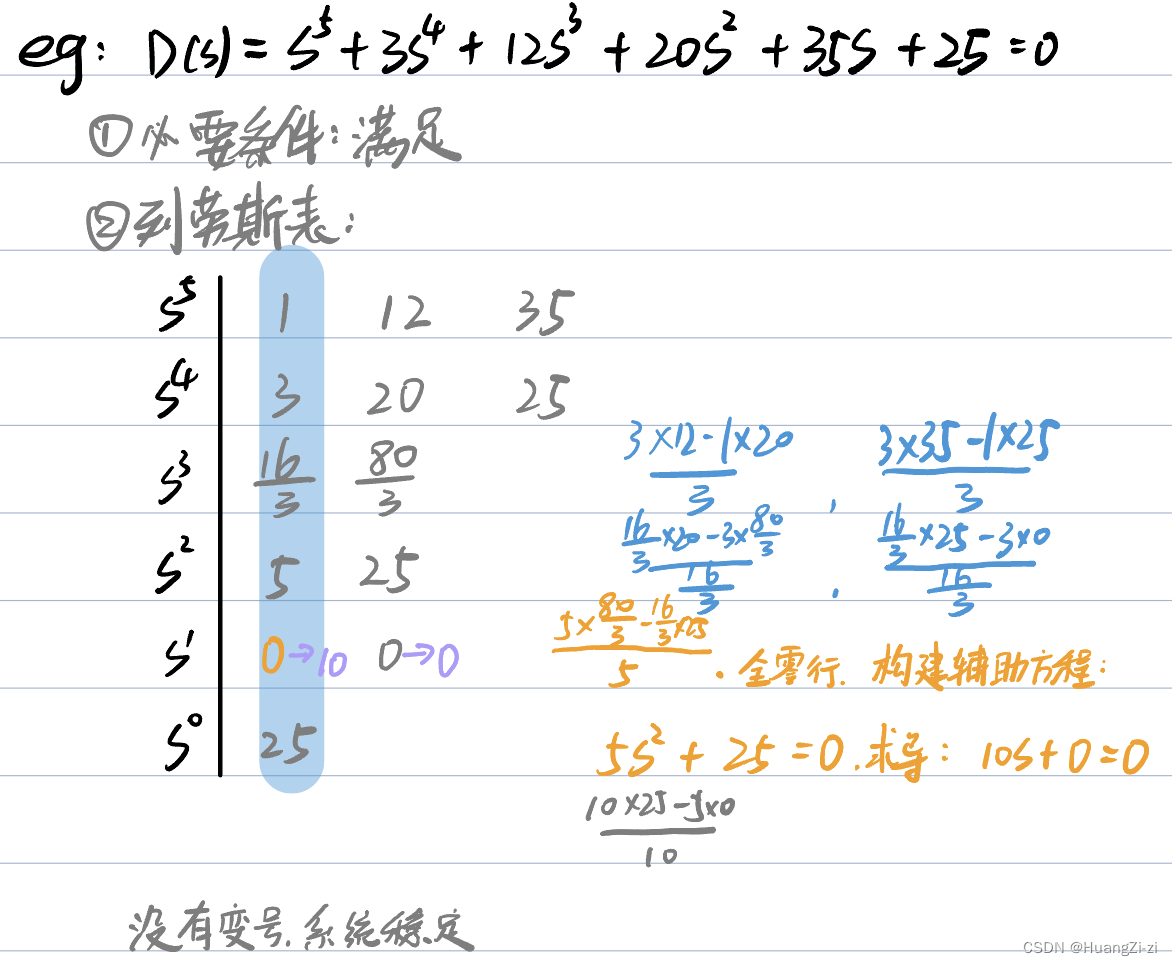### 问题辨析

1. 系统稳定性是系统自身的属性，与输入的类型、形式无关
2. 系统是否稳定，只取决于闭环极点，与闭环零点无关。（闭环零点影响动态性能，但不影响稳定性。闭环极点决定系统稳定性，也影响动态性能）
补：增加闭环零点：峰值时间靠前，超调量增大
增加闭环极点：峰值时间靠后，超调量减小
3. 闭环系统稳定性与其开环是否稳定无关

# 稳态误差

## 误差与稳态误差的定义

1. 按输入端定义的误差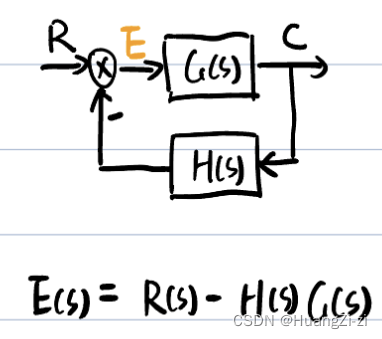2. 按输出端定义的误差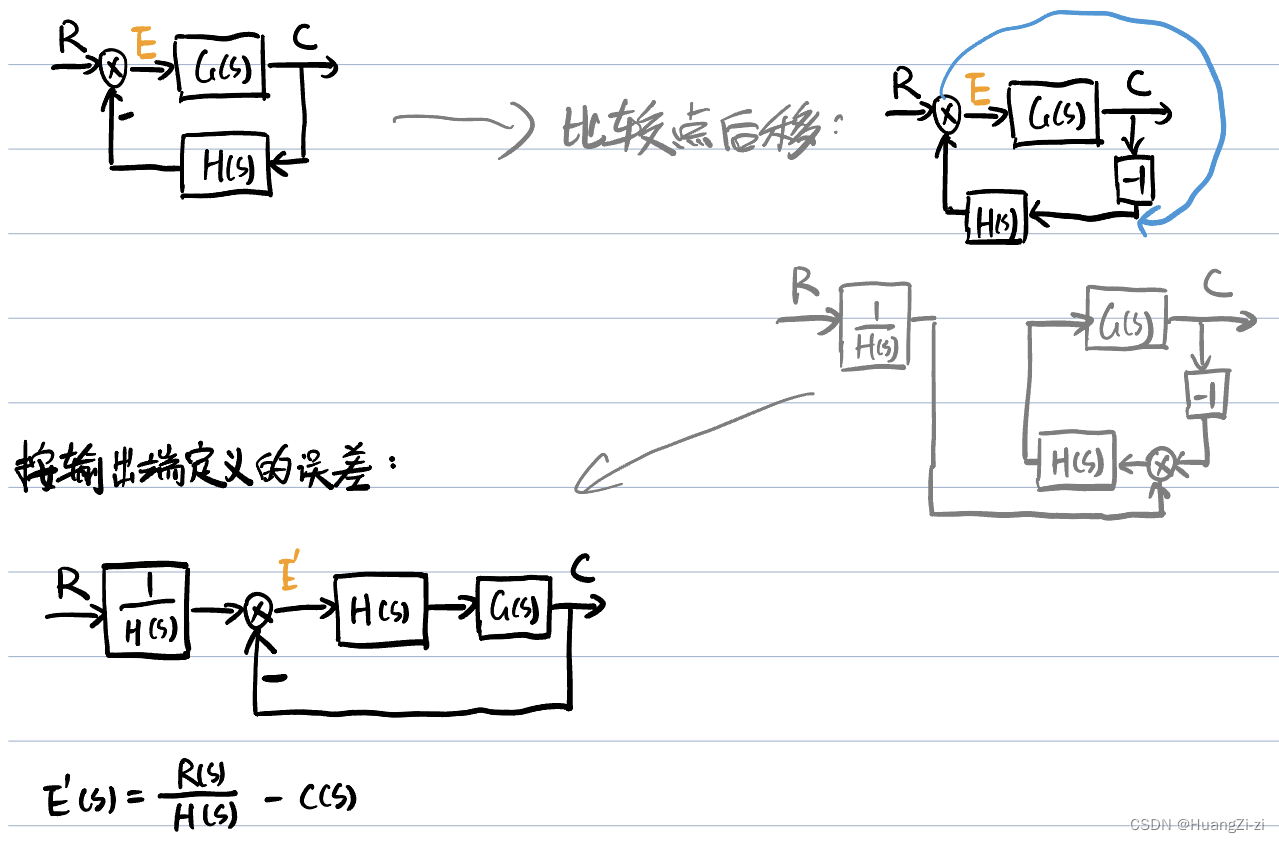两种定义本质上是一样的，如果再进一步推导，就有：
E′(s)=E(s)H(h)E'(s)=\displaystyle \frac{E(s)}{H(h)}

1. 稳态误差
误差传递函数：Φe=E(s)R(s)\Phi_e=\displaystyle \frac{E(s)}{R(s)}
e(t)=L−1[E(s)]=r(t)−c(t)e(t)=\mathscr{L}^{-1}[E(s)]=r(t)-c(t)
由于系统输出分为暂态分量和稳态分量，因此误差也分为暂态分量和稳态分量：
e(t)=ets(t)+ess(t)e(t)=e_{ts}(t)+e_{ss}(t)
ts->temporary state
ss->stable state，ess=lim⁡t→∞e(t)=e(∞)e_{ss}=\lim \limits_{t \rightarrow \infty}e(t)=e(\infty)
系统的稳态误差就是误差的稳态分量

## 计算稳态误差的一般方法

1. 判断系统稳定性「这一点非常重要，因为只有对稳定的系统研究稳态误差才有意义
2. 求误差传递函数「可以用梅逊公式快速得结果」
3. 用终值定理求稳态误差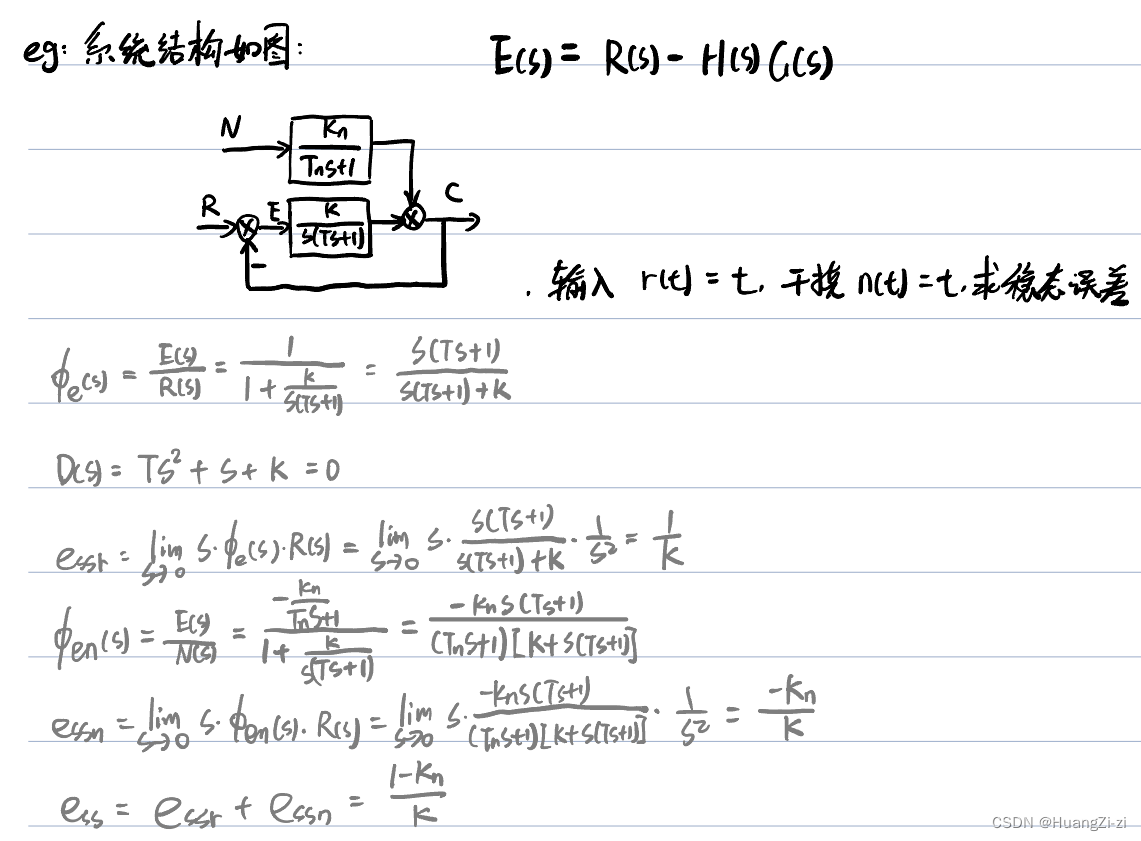## 静态误差系数法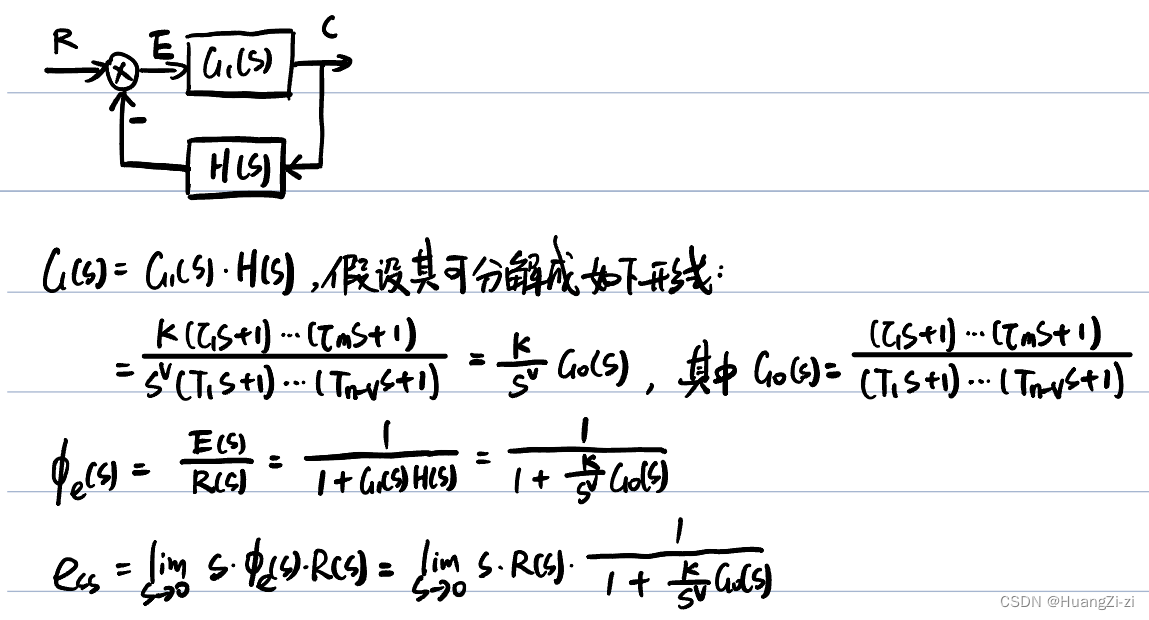G0化成尾1标准型所以K是开环增益
v是系统型别(就是一个分类标准，v=0叫做0型，v=1叫做1型)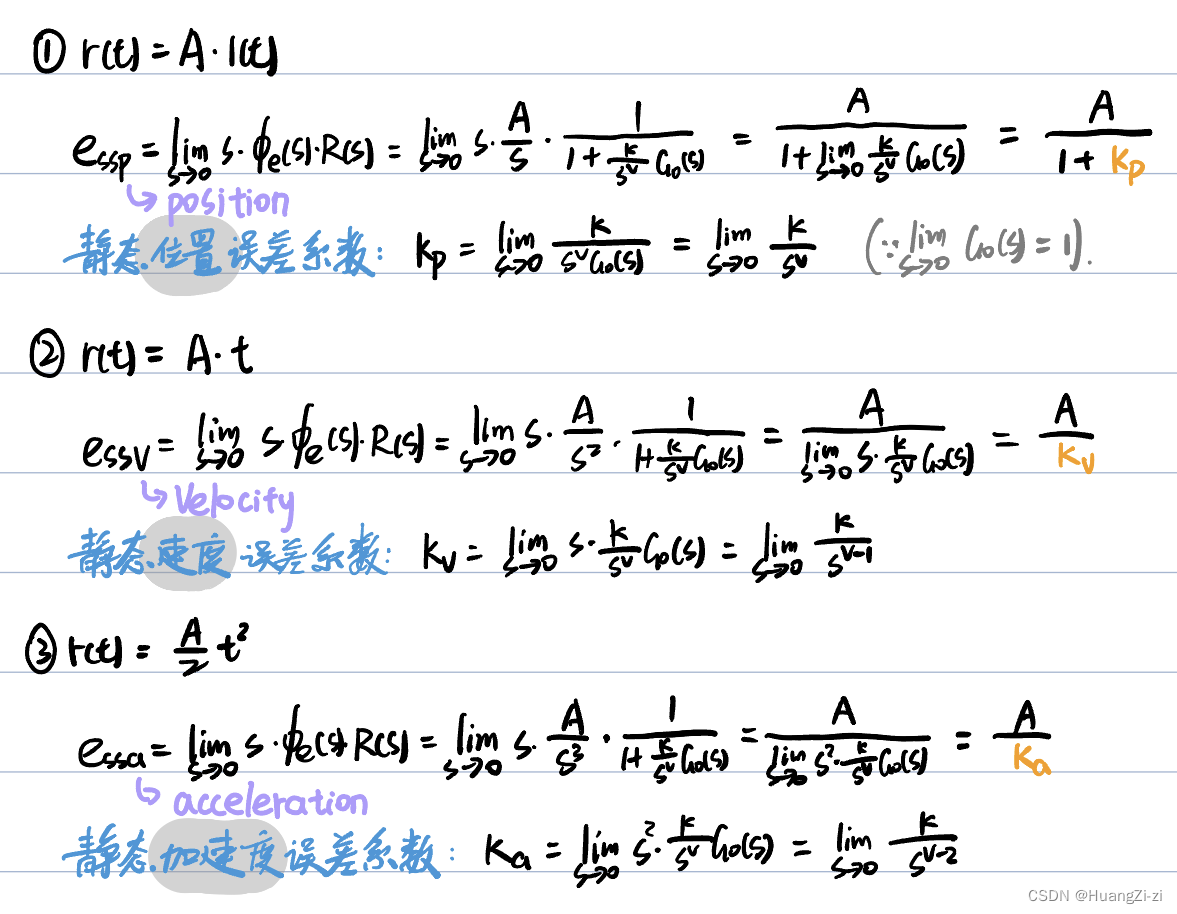0K00
1∞\inftyK0
2∞\infty∞\inftyK

0A1+K\frac{A}{1+K}∞\infty∞\infty
10AK\frac{A}{K}∞\infty
200AK\frac{A}{K}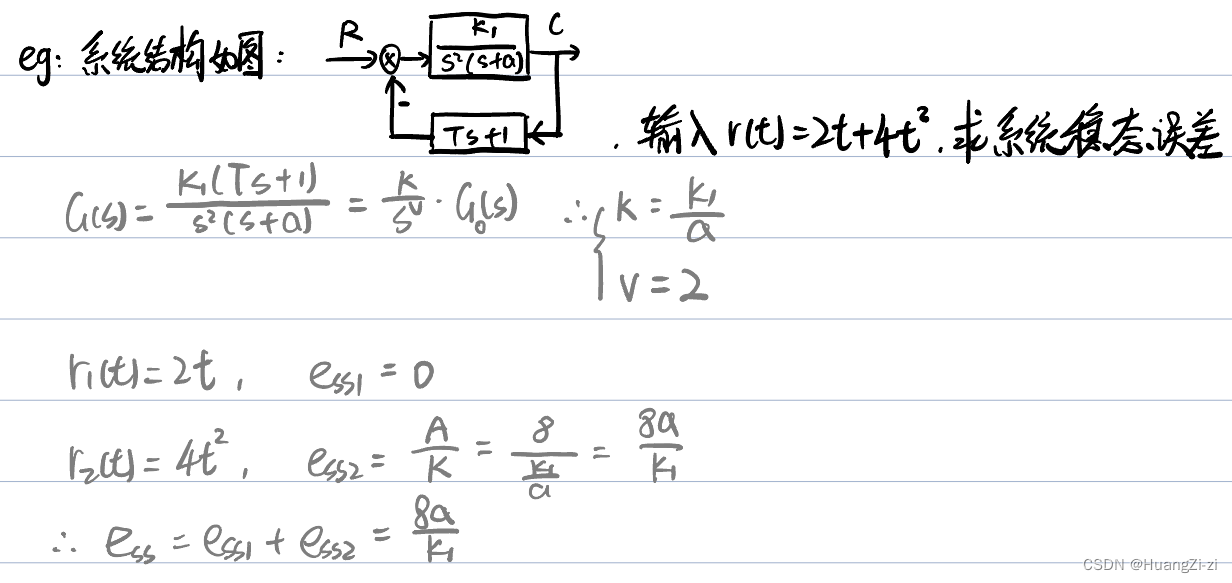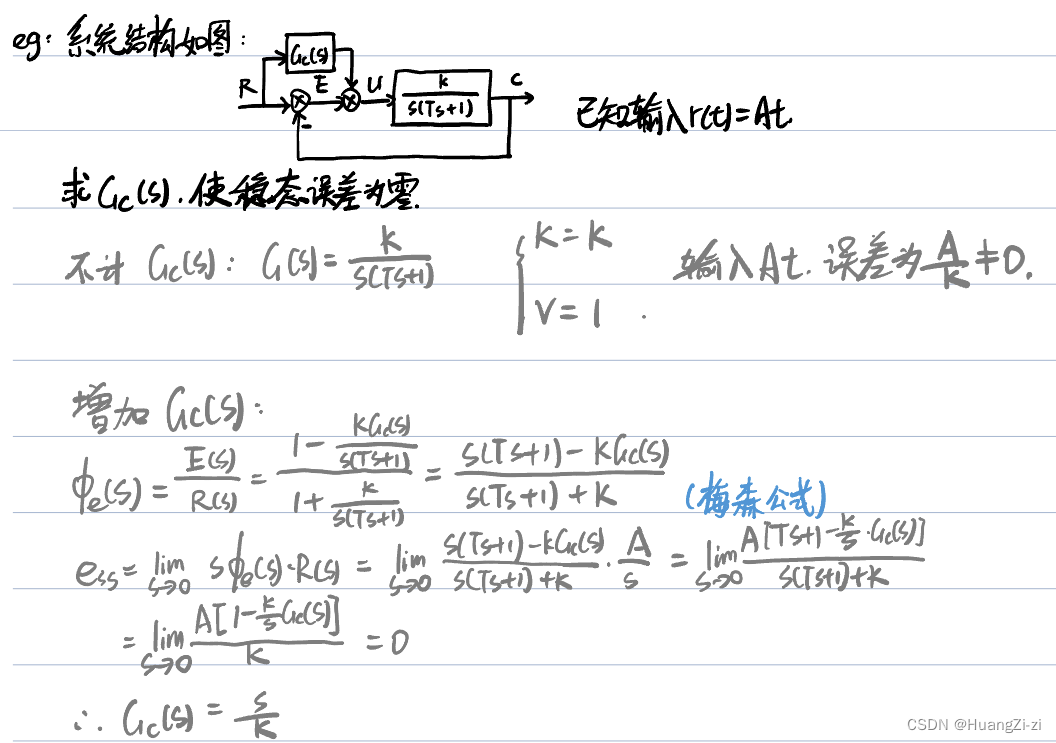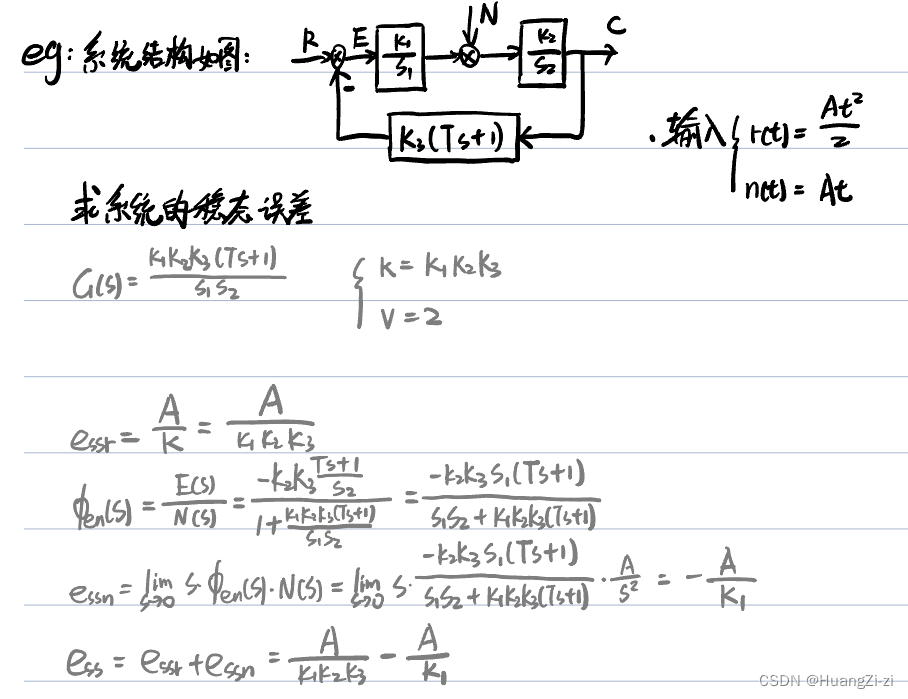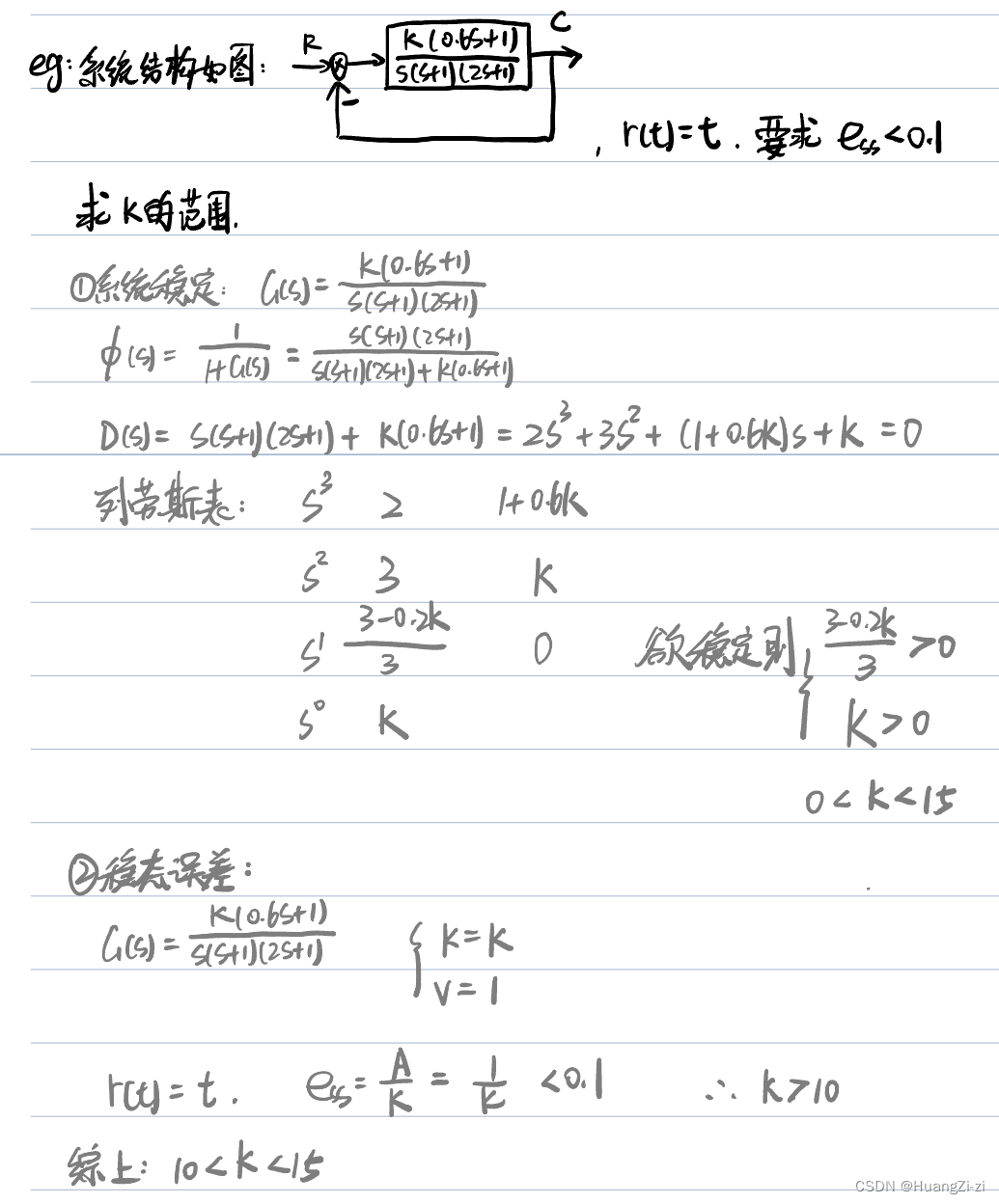## 动态误差系数法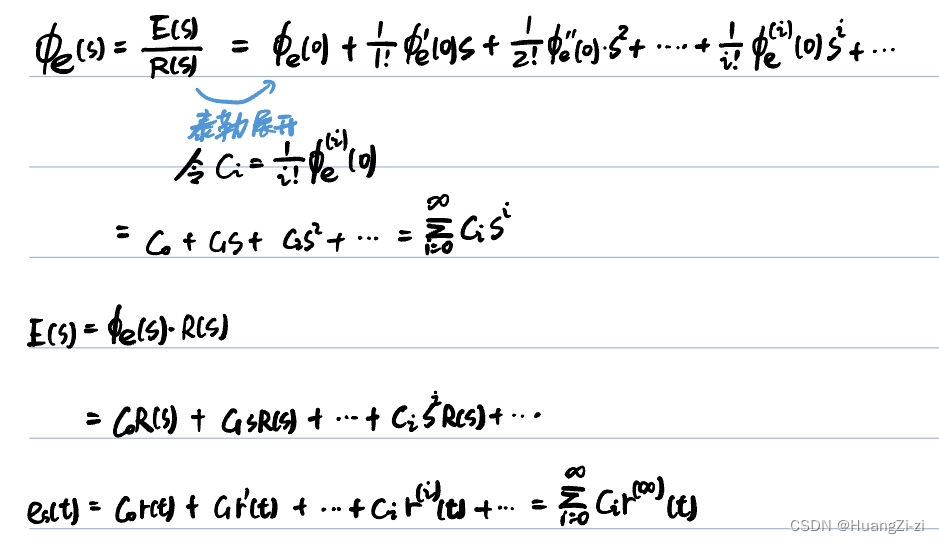（将开环传递函数按升幂排列才能除出级数的形式）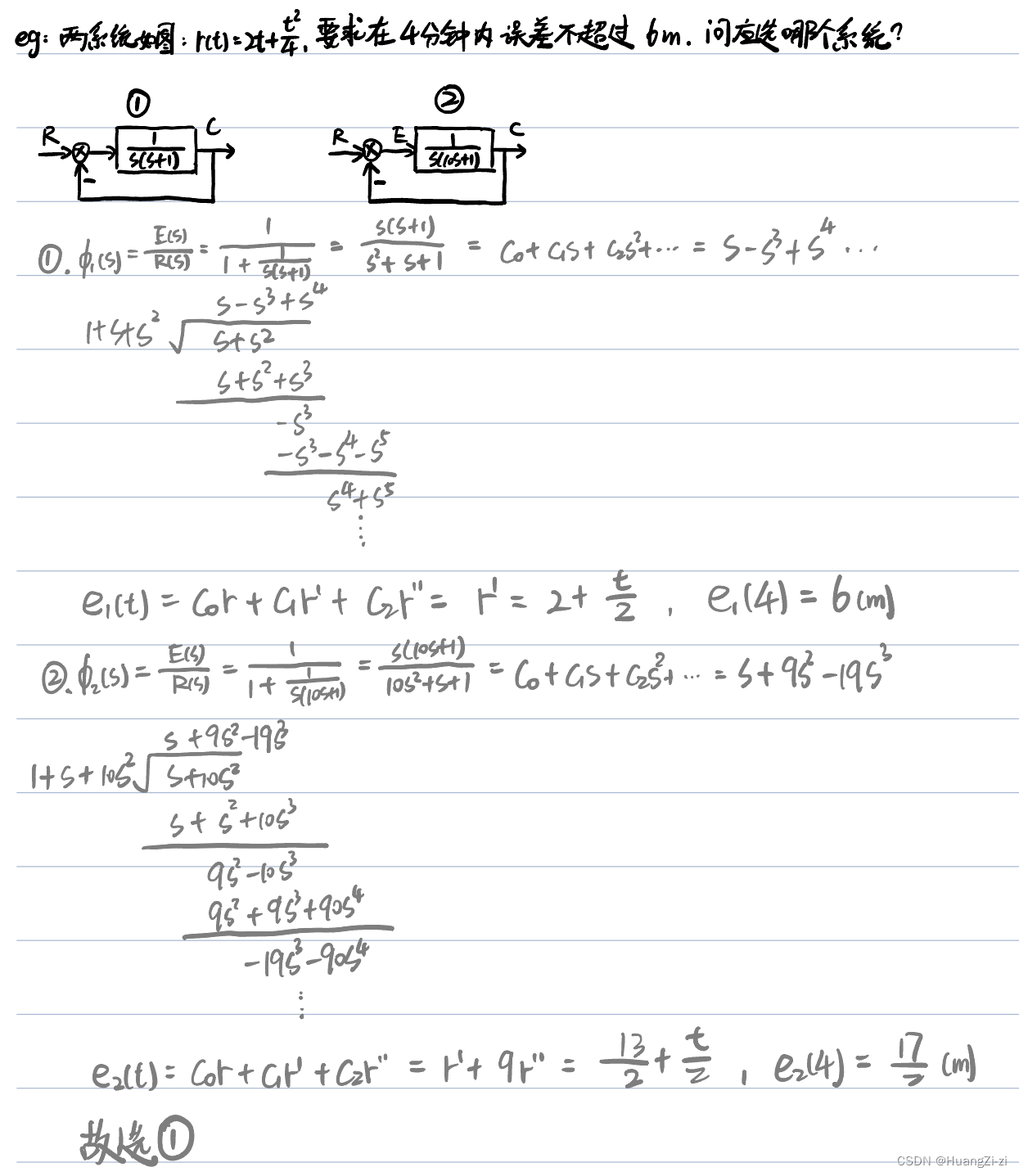## 扰动作用下的稳态误差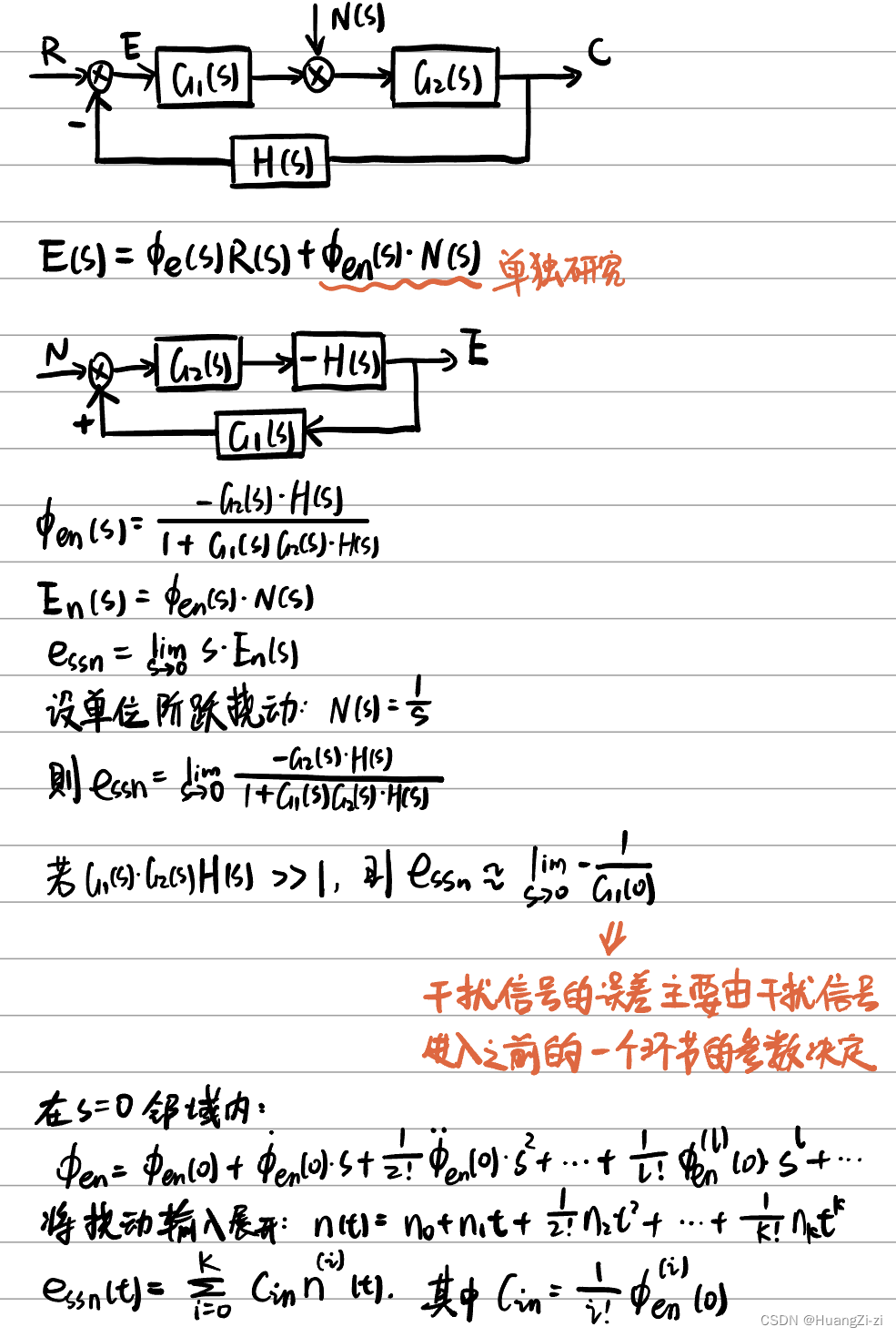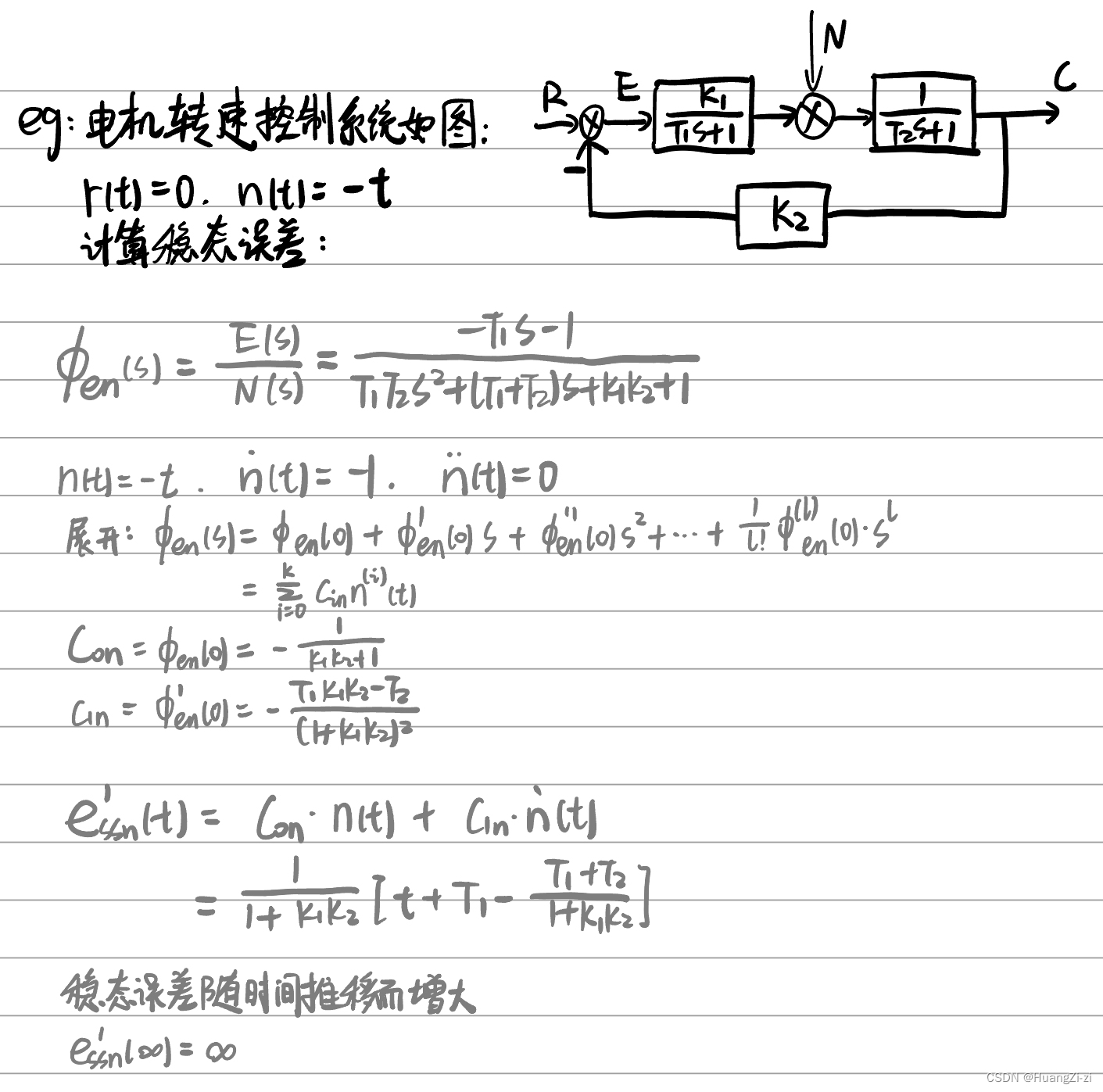# 时域校正

## 反馈校正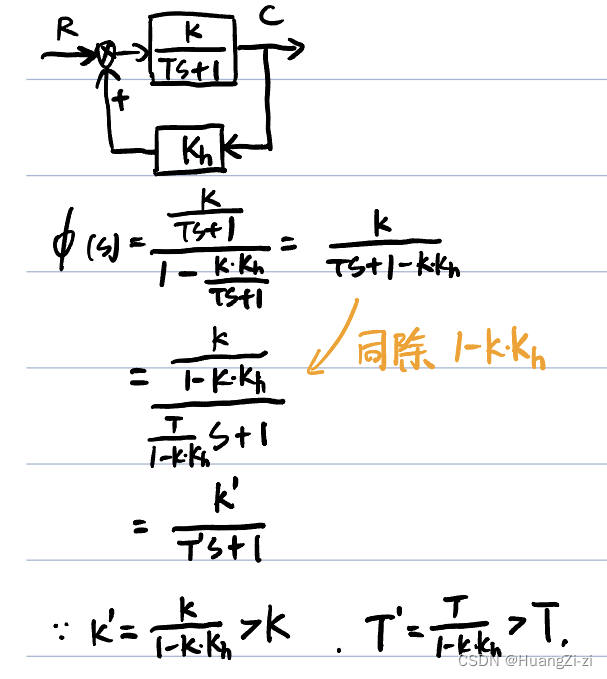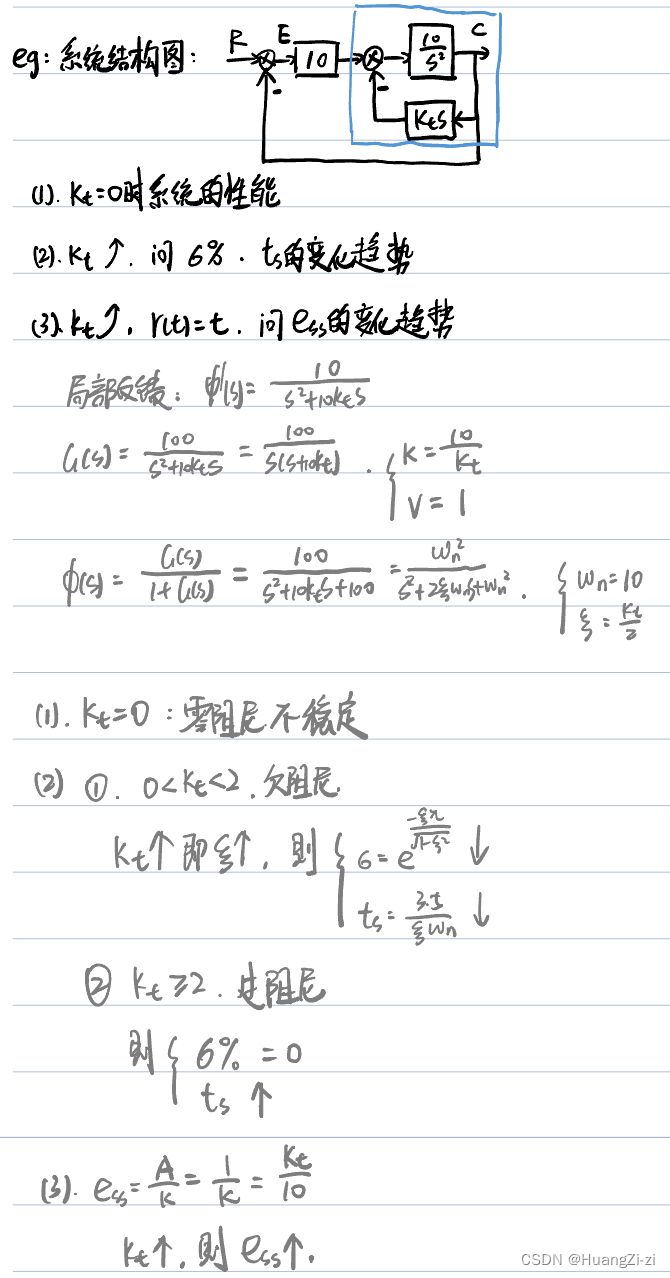## 复合校正